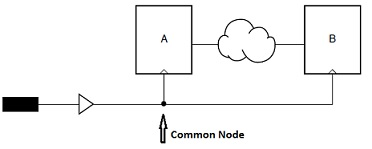# AR# 39744

|

## Description

Timing Analyzer takes Clock Skew into account when analyzing the setup/hold time.

How is Clock Skew calculated?

## Solution

Clock Skew is the delay difference between the source (SRC) clock path and the destination (DST) clock path.

The rough calculation is Clock Skew = DST clock delay - SRC clock delay.

In order to perform a worst case analysis, the timing tool uses a combination of max and min delay numbers for the SRC and DST clock paths.

Setup analysis uses min clock skew:

SKEWsetup = Min(DST clock delay) - Max(SRC clock delay)

Hold analysis uses max clock skew:

SKEWhold   = Max(DST clock delay) - Min(SRC clock delay)

However, the above equations introduce a pessimism factor to the clock skew, which comes from the common segment of the SRC and DST clock paths.

Performing (min delay - max delay) or (max delay - min delay) on the same path does not make sense and leads to over pessimistic clock skew.

So the common segment of the SRC and DST clock paths needs to be removed from the clock skew calculation.

Therefore, the common node (CN) where the two clock paths divert becomes the starting point to calculate the clock path delays.So the final equations of clock skew is as follows.

SKEWsetup = Min(DST clock delay starting from CN) - Max(SRC clock delay starting from CN)

SKEWhold   = Max(DST clock delay starting from CN) - Min(SRC clock delay starting from CN)